# We are examining a new project. We expect to sell 5,100 units per year at \$65...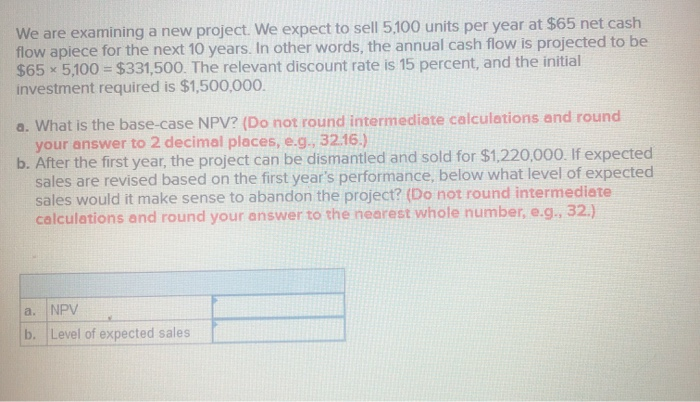We are examining a new project. We expect to sell 5,100 units per year at \$65 net cash flow apiece for the next 10 years. In other words, the annual cash flow is projected to be \$65 x 5,100 = \$331,500. The relevant discount rate is 15 percent, and the initial investment required is \$1,500,000. a. What is the base-case NPV? (Do not round intermediate calculations and round your answer to 2 decimal places, e.g., 32.16.) b. After the first year, the project can be dismantled and sold for \$1,220,000. If expected sales are revised based on the first year's performance, below what level of expected sales would it make sense to abandon the project? (Do not round intermediate calculations and round your answer to the nearest whole number, e.g., 32.) a. NPV b. Level of expected sales

Based on information available, we can answer the questions as follows:-

Requirement 1:-

The Net Present value is :- Present value of cash inflows - Present value of cash outflows

=\$331,500 * PVIFA(10 years , 15%) - \$1,500,000

=\$331,500 * 5.0188 - \$1,500,000

=\$1,663,732.2 - \$1,500,000

=\$163,732.2

Net Present Value = \$163,732(Rounded)

Requirement 2:

We would generally abandon the project if the cash flow from selling the equipment is greater than the present value of the future cash flows generated from the usage of equipment. In order to find that, we need go calculate the sales quantity where both of them are equal :-

\$1,220,000 = X * PVIFA(9 years, 15%) (\$65)

\$1,220,000 = X * 4.7716 (\$65)

\$1,220,000/(4.7716) (\$65) = X

X = \$1,220,000/310.154

X = 3,933.529

X = 3,934 units

It is beneficial if we abandon the project if the quantity is less than 3,934 units because the Net Present value of abandoning the project is greater than the Net present value of continuing with the project.

#### Earn Coin

Coins can be redeemed for fabulous gifts.

Similar Homework Help Questions
• ### We are examining a new project. We expect to sell 5,200 units per year at \$66...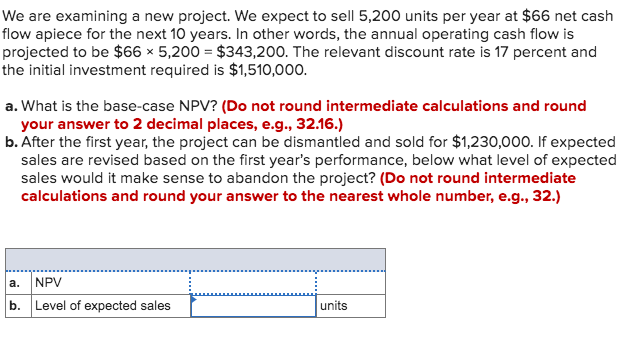We are examining a new project. We expect to sell 5,200 units per year at \$66 net cash flow apiece for the next 10 years. In other words, the annual operating cash flow is projected to be \$66 * 5,200 = \$343,200. The relevant discount rate is 17 percent and the initial investment required is \$1,510,000. a. What is the base-case NPV? (Do not round intermediate calculations and round your answer to 2 decimal places, e.g., 32.16.) b. After the...

• ### We are examining a new project. We expect to sell 6,200 units per year at \$76 net cash flow apiece for the next 10 years...

We are examining a new project. We expect to sell 6,200 units per year at \$76 net cash flow apiece for the next 10 years. In other words, the annual operating cash flow is projected to be \$76 × 6,200 = \$471,200. The relevant discount rate is 18 percent, and the initial investment required is \$1,730,000. a. What is the base-case NPV? (Do not round intermediate calculations and round your answer to 2 decimal places, e.g., 32.16.) NPV           \$...

• ### Problem 24-14 Abandonment Value (LO5] We are examining a new project. We expect to sell 5,400...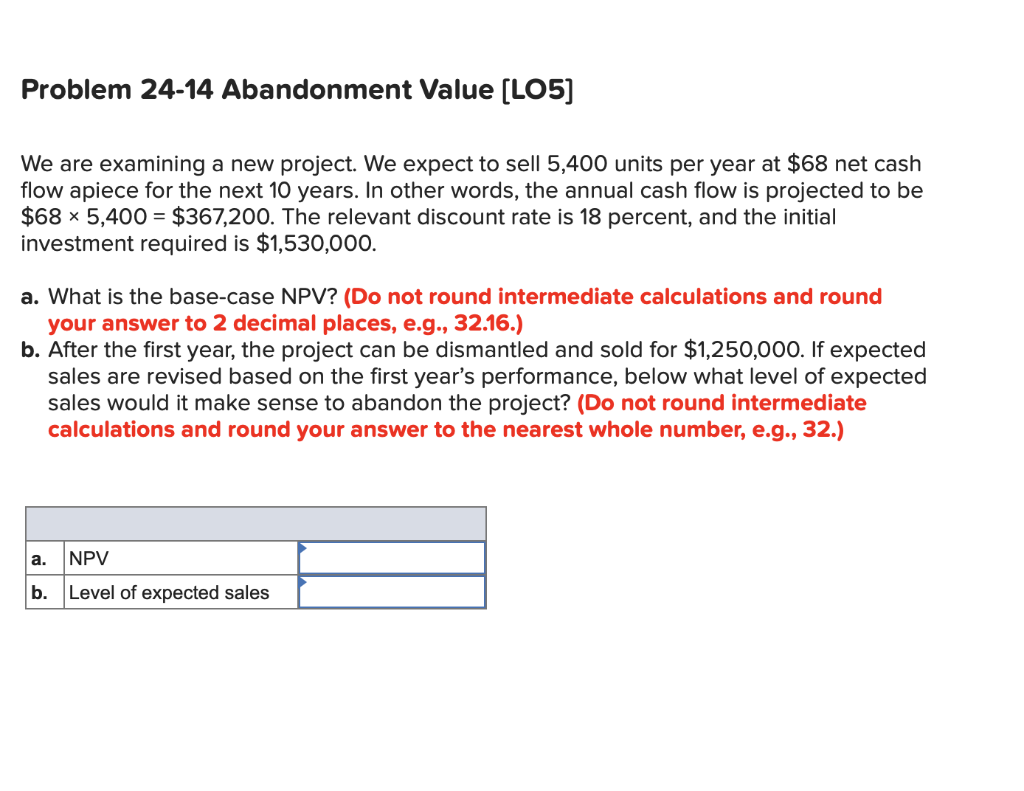Problem 24-14 Abandonment Value (LO5] We are examining a new project. We expect to sell 5,400 units per year at \$68 net cash flow apiece for the next 10 years. In other words, the annual cash flow is projected to be \$68 x 5,400 = \$367,200. The relevant discount rate is 18 percent, and the initial investment required is \$1,530,000. a. What is the base-case NPV? (Do not round intermediate calculations and round your answer to 2 decimal places, e.g.,...

• ### We are examining a new project. We expect to sell 6,900 units per year at \$63...

We are examining a new project. We expect to sell 6,900 units per year at \$63 net cash flow apiece for the next 10 years. In other words, the annual operating cash flow is projected to be \$63 × 6,900 = \$434,700. The relevant discount rate is 16 percent, and the initial investment required is \$1,800,000. After the first year, the project can be dismantled and sold for \$1,670,000. Suppose you think it is likely that expected sales will be...

• ### We are examining a new project. We expect to sell 5,800 units per year at \$72...

We are examining a new project. We expect to sell 5,800 units per year at \$72 net cash flow apiece for the next 10 years. In other words, the annual operating cash flow is projected to be \$72 × 5,800 = \$417,600. The relevant discount rate is 15 percent, and the initial investment required is \$1,690,000. After the first year, the project can be dismantled and sold for \$1,520,000. Suppose you think it is likely that expected sales will be...

• ### We are examining a new project. We expect to sell 6,200 units per year at \$76...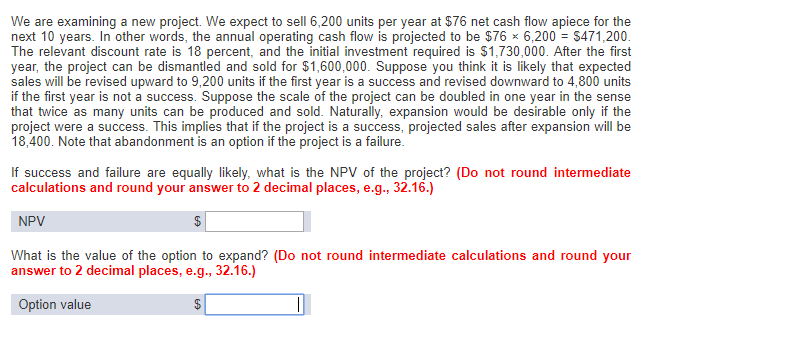We are examining a new project. We expect to sell 6,200 units per year at \$76 net cash flow apiece for the next 10 years. In other words, the annual operating cash flow is projected to be \$76 x 6,200 = \$471,200. The relevant discount rate is 18 percent, and the initial investment required is \$1,730,000. After the first year, the project can be dismantled and sold for \$1,600,000. Suppose you think it is likely that expected sales will be...

• ### We are examining a new project. We expect to sell 5,300 units per year at \$67...

We are examining a new project. We expect to sell 5,300 units per year at \$67 net cash flow apiece for the next 10 years. In other words, the annual cash flow is projected to be \$67 × 5,300 = \$355,100. The relevant discount rate is 16 percent, and the initial investment required is \$1,520,000. After the first year, the project can be dismantled and sold for \$1,240,000. Suppose you think it is likely that expected sales will be revised...

• ### We are examining a new project. We expect to sell 5,200 units per year at \$66...

We are examining a new project. We expect to sell 5,200 units per year at \$66 net cash flow apiece for the next 10 years. In other words, the annual cash flow is projected to be \$66 × 5,200 = \$343,200. The relevant discount rate is 17 percent, and the initial investment required is \$1,510,000. After the first year, the project can be dismantled and sold for \$1,230,000. Suppose you think it is likely that expected sales will be revised...

• ### We are examining a new project. We expect to sell 5,200 units per year at \$66...

We are examining a new project. We expect to sell 5,200 units per year at \$66 net cash flow apiece for the next 10 years. In other words, the annual operating cash flow is projected to be \$66 × 5,200 = \$343,200. The relevant discount rate is 17 percent, and the initial investment required is \$1,510,000. After the first year, the project can be dismantled and sold for \$1,230,000. Suppose you think it is likely that expected sales will be...

• ### We are examining a new project. We expect to sell 6,100 units per year at \$75...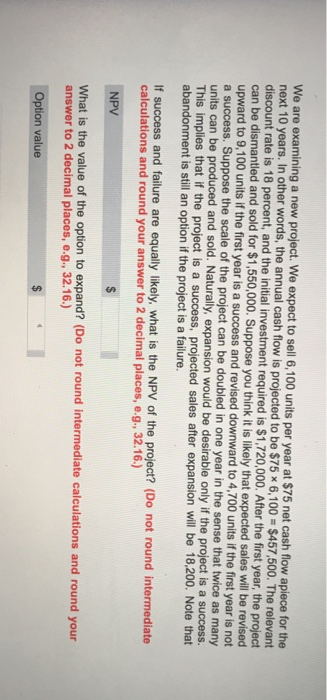We are examining a new project. We expect to sell 6,100 units per year at \$75 net cash flow apiece for the next 10 years. In other words, the annual cash flow is projected to be \$75 x 6,100 \$457,500. The relevant discount rate is 18 percent, and the initial investment required is \$1,720,000. After the first year, the project can be dismantled and sold for \$1,550,000. Suppose you think it is likely that expected sales will be revised upward...

Free Homework App